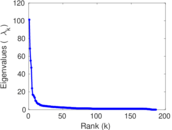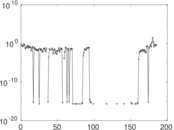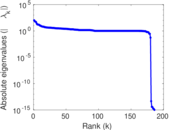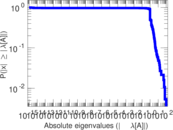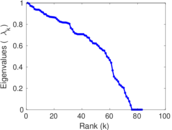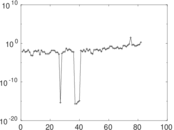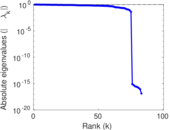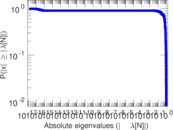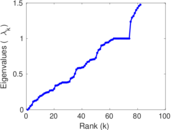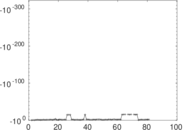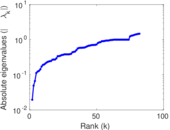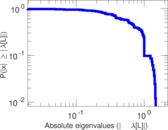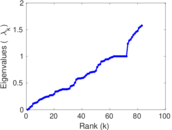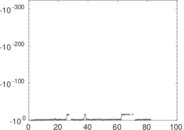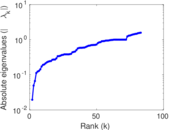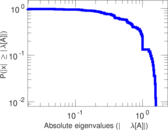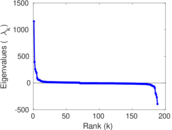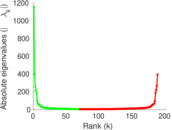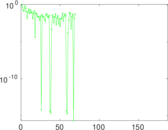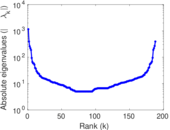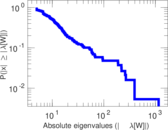# Wikibooks edits (be)

This is the bipartite edit network of the Belarusian Wikibooks. It contains users and pages from the Belarusian Wikibooks, connected by edit events. Each edge represents an edit. The dataset includes the timestamp of each edit.

 Code `bbe` Internal name `edit-bewikibooks` Name Wikibooks edits (be) Data source http://dumps.wikimedia.org/ AvailabilityDataset is available for download Consistency checkDataset passed all tests Category Authorship network Dataset timestamp 2017-10-20 Node meaning User, article Edge meaning Edit Network formatBipartite, undirected Edge typeUnweighted, multiple edges Temporal dataEdges are annotated with timestamps

## Statistics

 Size n = 1,160 Left size n1 = 188 Right size n2 = 972 Volume m = 2,411 Unique edge count m̿ = 1,288 Wedge count s = 105,810 Claw count z = 11,482,972 Cross count x = 1,044,486,615 Square count q = 2,828 4-Tour count T4 = 450,212 Maximum degree dmax = 969 Maximum left degree d1max = 969 Maximum right degree d2max = 159 Average degree d = 4.156 90 Average left degree d1 = 12.824 5 Average right degree d2 = 2.480 45 Fill p = 0.007 048 42 Average edge multiplicity m̃ = 1.871 89 Size of LCC N = 872 Diameter δ = 11 50-Percentile effective diameter δ0.5 = 3.238 24 90-Percentile effective diameter δ0.9 = 5.532 34 Median distance δM = 4 Mean distance δm = 3.709 06 Gini coefficient G = 0.708 718 Balanced inequality ratio P = 0.221 070 Left balanced inequality ratio P1 = 0.138 117 Right balanced inequality ratio P2 = 0.306 927 Relative edge distribution entropy Her = 0.807 583 Power law exponent γ = 4.727 03 Tail power law exponent γt = 2.561 00 Tail power law exponent with p γ3 = 2.561 00 p-value p = 0.000 00 Left tail power law exponent with p γ3,1 = 1.901 00 Left p-value p1 = 0.600 000 Right tail power law exponent with p γ3,2 = 3.921 00 Right p-value p2 = 0.254 000 Degree assortativity ρ = −0.214 984 Degree assortativity p-value pρ = 6.220 67 × 10−15 Spectral norm α = 101.217 Algebraic connectivity a = 0.019 450 8 Spectral separation |λ1[A] / λ2[A]| = 1.473 41 Controllability C = 791 Relative controllability Cr = 0.687 228

## Plots

### Fruchterman–Reingold graph drawing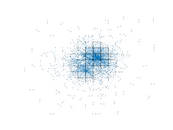### Degree distribution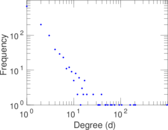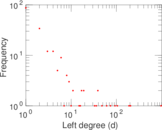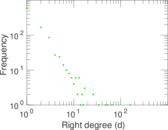### Cumulative degree distribution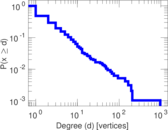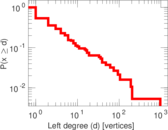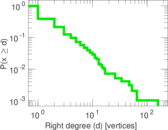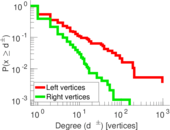### Lorenz curve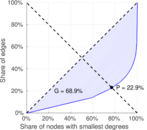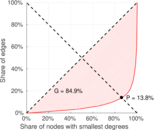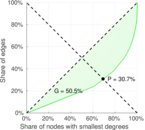### Spectral distribution of the adjacency matrix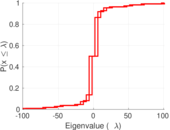### Spectral distribution of the normalized adjacency matrix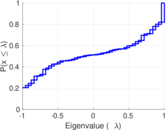### Spectral distribution of the Laplacian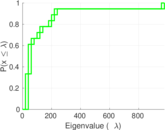### Spectral graph drawing based on the adjacency matrix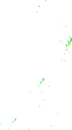### Spectral graph drawing based on the Laplacian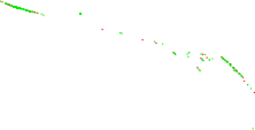### Spectral graph drawing based on the normalized adjacency matrix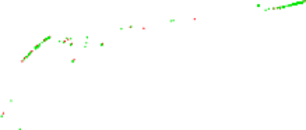### Degree assortativity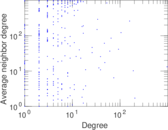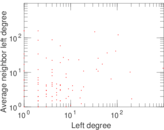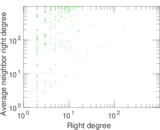### Zipf plot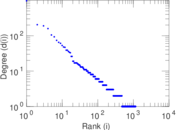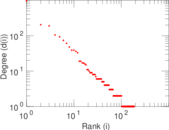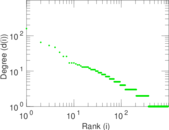### Hop distribution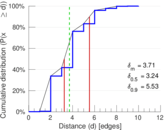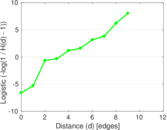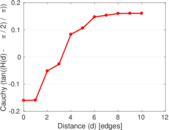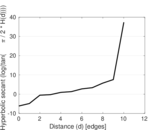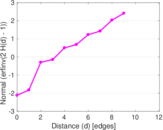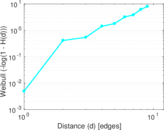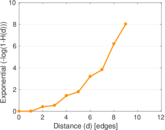### Double Laplacian graph drawing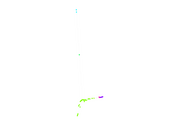### Delaunay graph drawing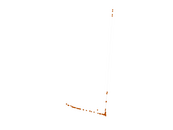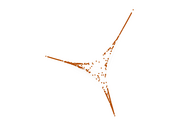### Edge weight/multiplicity distribution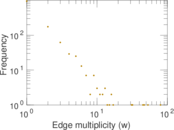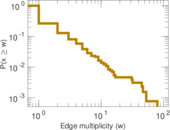### Temporal distribution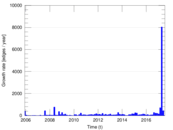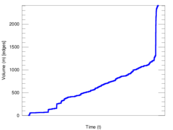### Temporal hop distribution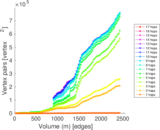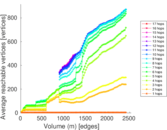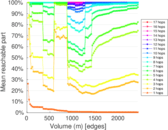### Diameter/density evolution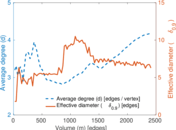### Matrix decompositions plots